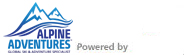TOLL-FREE 1.800.755.1330

## References

The metric system of measurement is used in Europe and Canada. Here are a few useful conversions:

1 kilometer (km) = 0.6 mile
1 mile = 1.6 kilometers
1 meter (m) = 3-1/4 feet
1 kilogram (kg) = 2.2 pounds
100 grams = 1/4 pound (approximately)
500 grams = 1.1 pound
1 liter (l) = 1 quart (approximately)

Temperatures are quoted in Celsius degrees. Approximate equivalents are:

0° Celsius = 32° Fahrenheit
5° C = 41° F
10° C = 50° F
15° C = 59° F
20° C = 68° F